Next: Orbits of Galaxies in Up: Orbits of Galaxies Previous: N-body Solution of the

# First Evaluation of Optimizing Methods

In Section 4.3, several methods for optimization of n-dimensional functions were presented, and some of their properties were discussed. One conclusion to be drawn is that the efficiency may vary quite considerably from one type of method to another. For small systems, the methods including the second derivative are usually thought to be preferred, while the methods only needing the first derivate are usually best suited when the number of variables is large. The reason for the reluctance of accepting these rather stringent observations from is that the behavior of an optimizing method is not only dependent on the size of the system, but also other characteristics, like scaling. In this section, I will therefore apply all the methods from Section 4.3 to the same, rather small system of only 120 unknown variables. One should expect the optimizing methods of category (3) from pageto be the ones best suitable, but, as the test in this section will show, this is not necessarily the case.

The system mentioned, for which the optimizing methods will be tested, is the same as in Subsection 5.1.2, i.e. the eight galaxies given in Table 5.1. The motivation for choosing this particular system follows: Firstly, the number of particles is moderate, reducing the number of variables to be determined, and thereby keeping the computing time on a reasonable level. Secondly, the system is thought to be cosmologically young, meaning the galaxies are approaching each other for the first time (with the possible exception of NGC 6822 P-90). This assures the crossing time to be less than the Hubble time, reducing the possibility of a multivalued solution. This is also the reason for not considering galaxies closer than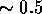Mpc to the MW/M31 pair, which, most probably, are gravitationally bound to either of these two galaxies. Finally, the system has been thoroughly studied before, e.g. by P-89,P-90, thereby presenting us with the characteristics of the solutions.

The choice of cosmology for this system will also be the same as in Subsection 5.1.2: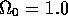and h=0.84, whereupon the same mass of M31 is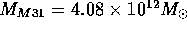. There is no other motivation for choosing this particular cosmology other than for the sake of comparison with the results of P-89 and Subsection 5.1.2.

The system of mass tracers may be the same, but there is one small, but yet distinctive difference, which will not affect the solutions, but most likely the convergence to these solutions. This difference lies in the choice of trial functions. In Subsection 5.1.2 and in P-89, the trial functions in equation (3.22) were applied, while the Bernoulli series from (3.23) will be used here. The change introduced by the more complex Bernoulli series is that the scaling of the system is considerably improved. A way to visualize this is to make use of the condition number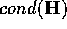of the Hessian matrix at the solution point, which is a measure for the quality of the scaling. For a system to be characterized as well scaled, this number has to be in the immediate neighbourhood of unity. Even with a value of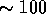, for which the matrix is not particularly ill-conditioned, the rate of convergence can be extremely slow. The condition number for the system treated in Subsection 5.1.2, using the trial functions from (3.22), was as large as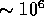--the slow convergence in that system could therefore be anticipated. A considerable improvement in convergence can be expected when applying the Bernoulli series 3.22) which reduces the condition number to about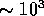. As we shall see in the case of the Steepest Descent method, this is indeed the case. The number of trial functions will be the same as in Subsection 5.1.2, namely N=5.

The evaluation of the optimizing methods for the type of problems treated in this thesis is based on a number of criteria, the most important being speed. The method's ability to drive the action to a stationary point as effectively as possible is essential to the AVP, at least when treating large systems of mass tracers; i.e. 20 and more. Therefore, the methods are timed, using the CPU-time. Another point of comparison is accuracy. For small numbers of N in the parameterization of the orbits in equation (3.21), the accuracy is initially poor and it is therefore unnecessary to drive the solution to high accuracy. However, if the truncation is relatively small, say N;SPMgt;20, the stationary values have to be determined to a comparative accuracy; i.e. if not as far as machine accuracy, at least close to it. Therefore, the testing also includes a check of the method's ability to locate the solution to high accuracy. The termination time is set to be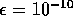, giving an accuracy in the positions to at least the eighth decimal digit, which is quite excessive when the truncation in the orbits (3.21) is as large as it is here (N=5). In comparison, the machine accuracy for the choice of variables in equation (5.3) is.

The last criterion, but not necessarily the least important, is the method's ability to locate different stationary values. P-90 showed that the Newton-Raphson method is capable of finding saddle points that are valid solutions to the equations of motion. To test the method's capability of finding different solutions for different starting points, each method was run for 100 different starting points, equally spaced between 0 and 10. Running the same program such a large number of times also gives the opportunity to get an average value for the computing time spent by the methods in reaching the solutions to given accuracy.

The results of the tests are given in Table 5.3. A description of the presentation follows. In Section 5.1, it was seen that optimization methods may have different implementations, e.g. different stepsize algorithms, all of which will be under trial. The type of optimizing method and the type of method for the determination of the stepsize will be given in column (1) and (2) in Table 5.3, respectively. In the remaining columns, the following are given: (3) a rough average of the number of iterations for the 100 different runs, (4) the time spent for the average value of iterations in column (3), (5) an estimate of the number of iterations per second in CPU-time, and, finally, (6) the number and type of allowed solutions found.

Let us now get to the actual testing, which, hopefully, will enable us to determine which optimizing methods is the most efficient for the rather small system considered in this section. A description of the test of each method with all the different implementations follows:

Method of Steepest Descent

As seen in Subsection 4.3.1, the Steepest Descent method is a very simple and stable method, but has a major drawback in having slow convergence--for a well scaled system its convergence is at best linear. We saw in Subsection 5.1.2 that the convergence indeed was slow, especially when applied to the rather poorly scaled systems considered there. As seen earlier in this section, the application of the Bernoulli series (3.23) gives improved scaling, which, as we shall see shortly, results in a considerably faster convergence of the Steepest Descent method. Another part of the scheme that is important for the convergence, is the use of a stepsize determiner. As seen in Subsection 4.3.1, one has the choice between a linear search and a method where the stepsize is solely being determined by the criterion that the action is to decrease at each iteration. (The latter method will henceforth be referred to as the the ``crude'' stepsize determiner.) Both methods have been tried here.

The application of the Bernoulli shaped trial functions resulted in a substantial reduction in computing time. While the Steepest Descent method using the trial function in (3.22) spent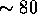seconds CPU-time in determining the solution to an accuracy of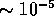in Subsection 5.1.2, using the Bernoulli trial functions located the solution inCPU-seconds on an average over the 100 different runs, then to an accuracy of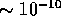(both using the crude stepsize determiner). There was little sign of the slowing down of the convergence as observed in Subsection 5.1.2.

The results of the test of the Steepest Descent are given in Table 5.3. It is worth noting that the use of linear searches does not result in any considerable faster convergence, opposed to the crude variant. The reduction of the number of iterations is not large enough to compensate for the computational cost of the linear searches. The resulting computing time is about three times larger than for the implementation using the crude stepsize determiner.Table 5.3: Comparison of the differnt optimizing methods for an eight-galaxy system, with 120 variables, and termination set at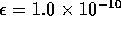. The columns are: (1) method, (2) stepsize algorithm, (3) average number of iterations for 100 different runs, (4) CPU-time in seconds used for the average value in column (3), (5) iterations per seconds in CPU-time, and (6) types of solutions located by the method.

The search for different solutions was unsuccessful; only one solution, a minimum point, was located.

The Conjugate Gradients method is, as shown in Subsection 4.3.2, generally known to have better convergence than the method of Steepest Descent, mainly due to more effective search directions. These directions are here either determined by the Fletcher-Reeves or the Polak-Ribière formula.

One of the problems with the Conjugate Gradients method is, as seen in Subsection 4.3.2, the possible loss of conjugacy during the iterations, the reason being roundoff errors, inaccurate linear searches, and the non-quadratic terms of the action. The problem with roundoff errors is tried mended by scaling the parameters as presented in equation (5.3), and by doing the calculations in double precision. But, as seen earlier, the condition number of the Hessian is still relatively large. We therefore have to expect a certain loss of conjugacy due to bad scaling.

The loss of conjugacy through linear searches is a problem that in theory can be avoided, but which in practise is inevitable. By using accurate linear searches, the conjugacy will be preserved from one iteration to another, but, unfortunately, such accurate linear searches do not exist for non-quadratic functions. The linear searches are then iterative, and the determination of the stepsize accurate enough to avoid loss off conjugacy would involve several evaluations of the function and its first derivative at each iteration. Hence, demanding too high accuracy in the determination of the stepsize can take up more computing time than what is gained by keeping the directional vectors conjugate. In other words, efficiency of the Conjugate Gradients method is a tradeoff between the accuracy of the linear searches and their computing time.

There are methods for handling the loss of conjugacy, making sure that the Conjugate Gradients method converges, and preferably, converges fast. One way of doing this is by restarting the iterative process. I have tried a couple of restarting schemes, such as restarting with a Steepest Descent step after n iterations (n denoting the number of variables) since the preceding restart has taken place, or by restarting when the following inequality fails Bert: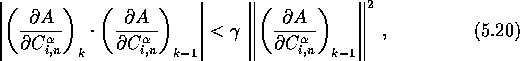where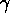is a fixed scalar with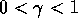. Equation (5.20) is a test of conjugacy; if the generated directions were conjugate, one would have.

The results of both using the Fletcher-Reeves and Polak-Ribière are given in Table 5.3, ``FP'' denoting the former and ``PR'' the latter. The secant method for the determination of the steplength was used in both cases. The difference in efficiency between the two methods is not great, the Polak-Ribière being somewhat slower than the Fletcher-Reeves formula. Based on the treatment in Section 4.3.2, this is somewhat unexpected. The Polak-Ribière has the ability to restart the iterative process when the directional vector is almost orthogonal to the gradient, the new direction being the direction of steepest descent, and should therefore have an advantage over the Fletcher-Reeves formula, which does not have this quality. But, by using the restarting scheme discussed above, the Fletcher-Reeves formula has been given a similar characteristic. The reason why the restarting scheme in equation (5.20) works better than the scheme incorporated in the Polak-Ribière formula is that the latter only restarts the iterative process when the directions are close to being non-conjugate, while with (5.20) on has the opportunity to restart the process before this occurs. The restarting scheme in (5.20) has therefore also been applied to the Polak-Ribière formula, and has improved the performance of this formula as well, but, as seen in Table 5.3, not enough to fully be comparable to the Fletcher-Reeves formula. The optimal value ofwas found to be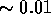.

Restarting after n iterations did not improve the convergence, on the contrary, in some cases it resulted in an increase in the number of iterations. This restarting scheme is therefore henceforth omitted.

Only one solution was located, it being a minimum point, the same solution that was found by the method of Steepest Descent.

The Newton-Raphson Method

The Newton-Raphson method is, as seen in Subsection 4.3.3, arguably the most complex of the optimizing methods, but also the one with the fastest convergence. Although it is both elaborate to calculate and to handle, the second derivative will provide the extra information on the action needed to locate the stationary values in an effective way.

For non-quadratic and non-linear functions, the rapid convergence of the Newton-Raphson method may be hindered by roundoff errors, non-quadratic terms, and a badly chosen starting point. As discussed in Subsection 4.3.3, this convergence problem can be mended by either using linear searches, backtracking, or a fixed value for the stepsize factor. Linear searches are generally found to be too computationally costly to give any gain in efficiency, but, will not be discarded before tested on the system treated here.

Backtracking, on the other hand, is usually known to be the best choice of stepsize determiner. The criterion for accepting the step length is DS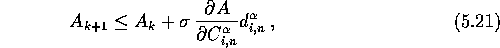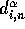being the direction of search. The parameter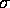is usually chosen close to zero, and in order to avoid the step size becoming too small, a cutoff of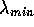is used. The subroutine used for the backtracking, lnsrch, is given by Press90.

P-94 used a different method in assuring the convergence of the Newton-Raphson method. He applied a fixed stepsize, which, initially is about 0.1, and approach unity sufficiently close to the solution, allowing the full Newton step. The stepsizes for different values of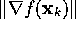used here areThis choice is not necessarily the optimal one when it comes to speed, but it does not ignore some of the stationary values which other choices may do. Giving the limit of the full Newton-step a value that is too small may result in the method only being able to locate one minimum point, while, on the other hand, by using a value that is too large will result in a multitude of solutions and excessive computing time.

The linear system equivalent to equation (4.18) is solved at each iteration by either Cholesky factorization or LU-factorization, depending on the Hessian matrix being positive or non-positive definite, respectively. The routines choldc, cholsl, ludcmp and lubksb given by Press90 were used.

A point made in Subsection 4.3.3 was that a non-positive definite Hessian matrix may lead to stationary solutions other than minimum points. As mentioned, P-90 showed that not only minimum points are valid solutions to the equations of motion; the saddle points were just as physically possible. Therefore, there are no constraint on the Hessian matrix being positive definite in this treatment. The validity of the different solutions are tested by the N-body code.

The results of the tests for the different stepsize algorithms are given in Table 5.3. (``Crude'' denotes, as before, the fixed stepsize method.) Surprisingly, the implementation using linear searches is the most efficient, using the the least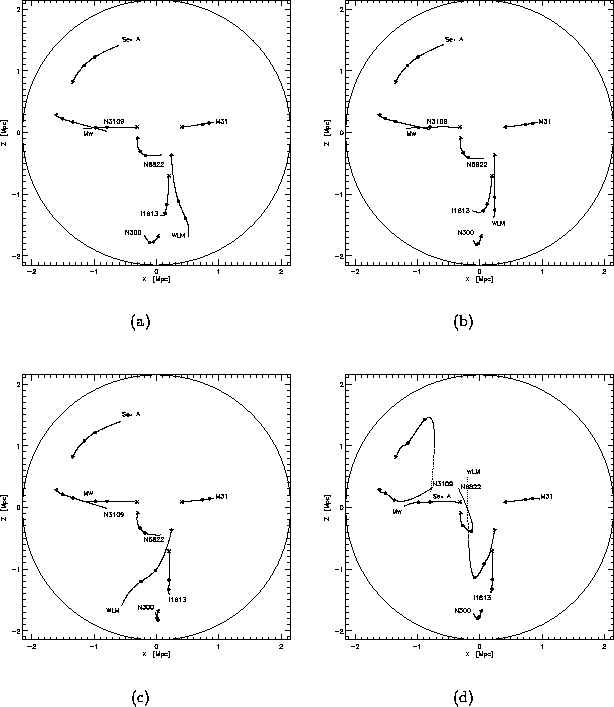Figure 5.3: Different types of solutions for 8 galaxies in the LG and the LN. (a) and (b) are minimum points, (c) is a saddle point and (d) is the orbits in a bad solution.

amount of iterations and spends the least amount of computing time. This may change for larger systems though, when the computations in the linear searches become more costly.

It should also be noted that the convergence is, as could be expected form theory, very fast close to the solution. Only a couple of iterations are needed to reduce the error from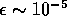to. For some of the solutions, the accuracy reached is as good as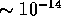even though the process is being terminated when the inequality in equation (5.4) is fulfilled. In other words, the accuracy can improve by as much as four factors of ten in one iteration close to the solutions. This is one of the more redeeming features of the Newton-Raphson method.

Several different solutions were located, both minima and saddle points. No maxima. Also, there where quite a few bad solutions; meaning they neither looks physically possible nor corresponds to the N-body orbits. The typical behavior of the orbits of these bad solutions is that some of the dwarf galaxies have short crossing time, and that some of the orbits seem to oscillate close to the present time. The search with backtracking encountered 26 different bad solutions, linear searches 3, and the crude stepsize algorithm only one. Examples of the different solutions, including a bad one, are given in Figure 5.3. Notice that the only difference between the three minimum points (Figure 5.1, 5.3(a), and 5.3(b)), is the orbits of some of the dwarf galaxies--the orbits of the two larger galaxies are barley changed. The saddle points have similar small deviations. Since the Newton-Raphson method is known to locate any type of solution, one can, as an initial guess, say that this eight galaxy system most probably has only three minimum points and at least five saddle points. A more extensive search would probably reveal more saddle points, but the number of minimum points would most likely stay the same.

The Secant Method

The Secant method has similarities to both the Conjugate Gradient method and the Newton-Raphson method. One could therefore expect it to have globally convergence, which, close to the solution is fast, preferably quadratic. This is not completely so. The method is globally convergent for the update formulas sustaining the positive definiteness of the Hessian at each iteration, but the convergence is rarely comparable to the Newton-Raphson method. The reason for this is that the buildup of the Hessian matrix for non-quadratic systems is not forced to be complete until an infinite number of iterations has been completed.

Both the DFP and the BFGS updating formulas were tried, and the initial guess of the Hessian matrix should then be positive definite. A common choice is, whereis the identity matrix, resulting in the initial step being in the steepest-descent direction. But, a problem with doing this, is that there is no consideration of the scale of the action. Therefore, a better choice would be DSwhich has been applied here.

As mentioned earlier, the convergence of the Secant method should be expected to be slower than for the Newton-Raphson method. The results in Table 5.3 confirms this, the number of iterations being larger for the Secant method. But, since there is no calculation of the second derivative, each iteration spends considerably less computing time. The Secant method therefore manage to take us to the solutions quicker than the Newton-Raphson method.

The difference in performance between the DFP and the BFGS updating formulas is very small when using linear searches, but is substantial when using backtracking: the DFP spending about ten times the iteration spent by BFGS implementation. The reason for this is that the performance of the DFP formula is very dependent on the stepsize being optimal, if not the conjugacy may be lost. Backtracking gives only a rough estimate of the stepsize.

It is confirmed here, what was stated in Subsection 4.3.4: the Secant method is only able to locate minima. But, for some reason, by using the linear search, only two minimum points were found, while using backtracking revealed three. I can not see any reason why using the linear search should fail to locate the third minimum point. A more extensive search may be able to reveal it.

A Hybrid Method

A hybrid of the two methods Steepest Descent and Newton-Raphson has also been tested, henceforth referred to as the Hybrid method. The reason for this is to test whether there is any gain in trying to combine the fast initial convergence of the Steepest Descent and the quadratic convergence of the Newton-Raphson method close to the solution. The implementation of each of these two methods which were found to work best for this Hybrid method, were the ones with linear searches.

The process is initiated by taking a step in the direction of steepest descent. In the following iterations the convergence of the Steepest Descent is tested, and when exhausted, the Newton-Raphson method takes over. This switch occurs when the following inequality fails: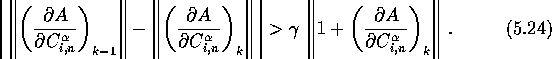The Hybrid method again returns to the Steepest Descent if this inequality later should be fulfilled. The value ofwas determined by trial and error to be 0.05, resulting in a rapid convergence, and the revelation of several different solutions. There are other choices forthat may improve the convergence even more, but then at the cost of the method's ability to locate different solutions. A total number of seven solutions were located, nine of them being bad ones. The results is given in Table 5.3.The test results in Table 5.3 for the optimization methods tried here are by no means conclusive, at least not when it comes to deciding which method is best in locating stationary values of the action. A test based on a larger and more realistic system will therefore be needed. In the next section, the methods that passed the test here will be tested on a system of 22 mass tracers in the LG and LN, the resulting number of variables being 660. Even though the test in this section has not given us a preferable method, it has at least excluded some of the implementations of some of the methods. I close this section by passing judgments on some of these implementations.

The method of Steepest Descent has shown itself to be as slow and inefficient as could be expected from the presentation in Subsection 4.3.1, at least when dealing with such a relatively bad-scaled system as the one considered here. The scaling of the system, and thereby the convergence, was greatly improved when going from using the trial functions in equation (3.22) to the Bernoulli distribution in (3.23), but it was not enough to make the method work satisfactory. Still, the method will not be discarded just yet--the speed of the method, especially when using the crude stepsize algorithm, can prove to be useful when dealing with larger systems. As seen in Table 5.3, the crude Steepest Descent method is superior to all the other methods when it comes to number of iterations per second of CPU-time, mostly due to it only needing the calculation of the first derivative at each iteration. The implementation using linear searches, on the other hand, has proven to be the least efficient optimizing method tried here. Its slow convergence is not being compensated by rapid iterations in the same acceptable manner as with the crude stepsize determiner. The method of Steepest Descent using linear searches will therefore not be considered in the remaining part of this thesis.

The Conjugate Gradients method was able to locate the solutions considerably faster than the method of Steepest Descent, which could be expected from the theoretical presentation in Subsection 4.3.2. Even though each iteration spent somewhat longer CPU-time than in the method of Steepest Descent, it spent a factor of fifty fewer iterations in locating the solutions. This resulted in the Conjugate Gradients method spending less than a 10of the time spent by the method of Steepest Descent in finding the optimized action.

By comparing the two different implementations of the Conjugate Gradients method in Table 5.3, there seem to be no reason in preferring one of the updating formulas over the other. The Fletcher-Reeves formula seem to be a somewhat better choice, but, with the general view from literature that Polak-Ribière is to be preferred for non-quadratic functions [e.g.][]Press,Bert in mind, it will not be given the final judgment before it has been submitted to further tests.

The Newton-Raphson method was, as could be expected, a very efficient optimizing method, at least for the relatively small system considered in this section. The convergence was very rapid, and the number of different solutions was large. Also, due to the very rapid convergence close to the solution, one is able to increase the accuracy of the solution considerably by only extending the search for a few more iterations. A minus with the method is, as discussed earlier, that each iteration is very slow due to the calculation and handling of the second derivative. This drawback will become substantial for large systems, so the final judgment on the method has to await the test in the next section.

The large number of solutions found by the Newton-Raphson method may be proven to be a double-edged sword. When locating several different minimum points and saddle points, it also frequently ends up at bad solutions. This is especially a problem while using the backtrack stepsize determiner. Therefore, it may be favorable to sacrifice the number of saddle points for fewer bad solutions by using the linear searches.

The Secant method proved to have somewhat slower convergence compared to the Newton-Raphson method. But, due to it not needing the calculation and inversion of the second derivative matrix, it spends less computing time. The choice between these two methods should therefore be obvious, but, since saddle points may be just as good solutions as the minima, the Newton-Raphson method still holds an advantage. But, if one is only interested in minimum points, the Secant method is probably the best choice of optimizer of all the methods tested here, with the possible exception of the Hybrid method. The only problem may be storage for systems with a very large number of variables--the Conjugate Gradients method or the Steepest Descent method may then be preferable.

Of the two updating formulas tested, DFP and BFGS, the latter was chosen for later use. This decision was based on three points: (i) the extremely slow convergence of the DFP formula using the backtracking determiner, (ii) there being no difference in convergence when using linear searches, and (iii) the general acceptance in literature of the BFGS over the DFP [e.g.][]Press,DS,GMW.

At last we have the Hybrid method, using the Steepest Descent initially, later ending up at the solution by the quadratic convergence of the Newton-Raphson method. This method proved to be one of the faster methods tested, and at the same time being able to locate the three minima, three saddle points, and an acceptable small number of bad solutions. This method may very well be the best choice of optimizing method. But, as with the Newton-Raphson method, it may be too computationally costly for a system with a large number of dimensions.

As seen, there are quite a few indications of which optimizing method is the best for the type, and the size, of system treated here. But, the picture may very well change when tested on a much larger system. Such a system will therefore be considered next.Next: Orbits of Galaxies in Up: Orbits of Galaxies Previous: N-body Solution of the

Trond Hjorteland
Mon Jul 5 02:59:28 MET DST 1999